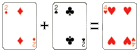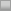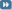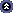Two Plus Two Poker ForumsRisk of Ruin Simulator and Calculator
 Register FAQ Search Today's Posts Mark Forums Read TwoPlusTwo.com

 Notices

 MTTSNG Discussion and analysis of MTTSNGs.08-30-2011, 08:11 PM #1 Sherman Carpal \'Tunnel   Join Date: Jun 2005 Location: Psychology Department Posts: 7,764 Risk of Ruin Simulator and Calculator Hi STT/MTT folks. I built a function that works in a free program (called R - you can download it at www.r-project.org) for simulating your risk of ruin. I have pasted the function below, but first let me explain a few things. Risk of Ruin (RoR hereafter) is the probability that an investor or gambler loses so much of his capital that he or she can no longer recover his or her losses (i.e. keep playing). The traditional risk of ruin formula is given as e-2 * WR * BR / Var where e is the constant 2.78128, WR is your expected win-rate (ROI), BR is your starting bankroll, and Var is the mathematical variance of results around your expected win-rate. You can find calculators for computing your risk of ruin using this formula online at websites like this one: http://www.poker-tools-online.com/riskofruin.html However, one problem with using such a formula is that your data meet certain assumptions. In particular, because the variance is used it assumes that your results are normally distributed. However, in MTTs (and perhaps in STTs) these assumptions are not necessarily met. So another method, one that is free of assumptions, to estimate your risk of ruin is needed. The function I distribute here provides us such a method. The function is listed here: Code: ```RoR.sim <- function(prizes, probs, startBR=100, winAmt=5*startBR, sims=1000) { if(sum(probs) != 1) {stop("Finish probabilities do not total 1.00. Please adjust input probabilities.")} if(length(prizes) != length(probs)) {stop("prizes and probs must be the same length (have the same number of elements).")} draws <- probs*10^(max(nchar(probs))-2) finishdist <- rep(prizes, draws) ROI <- sum(finishdist) / (-1*min(finishdist)*length(finishdist)) if(sims==FALSE) {tradRoR <- exp(-2*(sum(finishdist) / length(finishdist))*startBR/var(finishdist)) out <- rbind("ROI %"=ROI, "Traditional RoR"=tradRoR) colnames(out) <- "Results" return(out) } res <- rep(NA, sims) print("Simulating...please wait") for (i in 1:sims) { BR <- startBR if(i == .25*sims) {print("25% of sims complete.")} if(i == .5*sims) {print("50% of sims complete.")} if(i == .75*sims) {print("75% of sims complete.")} while(BR >= abs(min(prizes)) & BR < winAmt) { BR <- BR + sample(finishdist, 1, T) } res[i] <- BR } tradRoR <- exp(-2*(sum(finishdist) / length(finishdist))*startBR/var(finishdist)) out <- rbind(sims, ROI, tradRoR, sum(res < 1) / sims, sum(res >= winAmt) / sims) rownames(out) <- c("Sims", "ROI %", "Traditional Ruin Prob.", "Simulated Ruin Prob.", "Simulated Win Prob.") colnames(out) <- "Results" return(out) }``` Code: ``` #Example prizes <- c(48.571, 32.048, 18.6636, 12.2192, 9.7406, 7.2620, 4.7834, 3.2962, 1.8091, .9829, -1) probs <- c(.0071, .0069, .0068, .0067, .0067, .0066, .0066, .0065, .0064, .0590, .8807) RoR.sim(prizes, probs) RoR.sim(prizes, probs, startBR=40) # Maybe you only have a bankroll of 40 units # A headsup STT alternative # Note that this one uses numbers for a \$10.50 SNG for prizes. # But it still only has a bankroll of 100. # The number of simulations is adjusted as well. RoR.sim(prizes=c(20,-10.5), probs=c(.55, .45), sims=10000) # If you don't want the simulation, just the traditional RoR # calculation you can set sims=F. You can also give yourself # 100 BuyIns by changing the startBR to 100*10.5 RoR.sim(prizes=c(20,-10.5), probs=c(.55, .45), startBR=100*10.5, sims=F)``` Let me explain a bit about how the function works in its simplest form, and then more about the some of the options that make it more flexible. Logically the function asks for you to give it a vector that contains the prize distribution of a particular tournament (e.g. the 12/180 prize structure). However, you do not have to give it every possible prize, only the different prizes (e.g. if 10th-18th pay the same, you just list that amount once; likewise for when you don't cash--just list the amount of not cashing once). Next, the function asks you to give it a vector that contains the probability of ending up with each of the prizes listed in the vector of prizes (note: these vectors must be the same length). After that, the function will run on its own and return the results. What the function does is simulate you playing tournaments based on the prize and probability distributions your provided. It records how often you "win" (see below) or "ruin." It outputs your Return on Investment (ROI) given the distribution you provided, the number of simulations run, the traditional RoR result (following the traditional RoR formula listed above), the simulated Risk of Ruin probability, and the simulated win probability. That is the simplest level of operation. However, there are number of options you may wish to change from their defaults. The first is the startBR option. The startBR option contains the information about your starting bankroll. It defaults to 100 if you don't tell it anything, but you can specify startBR= to be whatever you want. The next option is the winAmt option. The winAmt option tells the function when to decide to stop playing and assume you have "won" (i.e. you won't go broke once you reach that point). By default it is set to 5 times the amount of your starting bankroll. Lastly, the sims option lets you decide how many simulations you want to run. Of course, running more simulations will result in more precise results, but the downside to simulations is that they take time. The function is built to tell you when 25%, 50%, and 75% of the simulations are completed (try making it run 10,000 simulations and then periodically click your mouse on the R console to see if it has updated). It is defaulted to run 1000 simulations. However, if you are in a real hurry and only want the traditional RoR formula, you can set sims= to FALSE and the function will quickly output your ROI and the traditional RoR formula result. If you are unfamiliar with R, I have sort of skipped over some things about what it means to "run" the function and things like that, so I will try to describe them briefly here. To use this function, copy and paste it into the R console. If everything went well, R should do nothing. Then try the examples (listed below the program code). If you run the line that starts with "prizes <- c..." R will store the information that follows it as a vector of prizes. So if you have your own prize distribution, you can replace the numbers inside the "c()" function with your own numbers...each separated by a comma. The same is true for the line that starts with "probs <- c..." Of note, in R comments start with a # tag. So you don't have to run the lines that start with a #. Those are just comments to the user for the examples. Then you can try the first example (or your own if you have the information) without changing any of the other options. After that, you can run the other examples as well. I'm happy to support this function for as long as I am around, so if you are using it and run into problems, feel free to send me a PM. I probably won't check back here that often (probably until the thread dies out), but feedback can be left here as well.08-30-2011, 09:50 PM #2 Grindation veteran   Join Date: Aug 2010 Location: At the top Posts: 2,077 Re: Risk of Ruin Simulator and Calculator 1st. will test tomorrow. looking forward to it08-30-2011, 11:57 PM #3 Sherman Carpal \'Tunnel   Join Date: Jun 2005 Location: Psychology Department Posts: 7,764 Re: Risk of Ruin Simulator and Calculator I found a couple of problems when using fractions that repeat forever as probabilities, so I made an adjustment (and a couple of other small ones). I would appreciate testing though because it late for me and I am afraid I might have some errors (either in terms of calculating traditional RoR or ROI). This adjustment means that it will treat finish probabilities that repeat past 6 decimals as rounded to 6 decimals. It is also important to note that under "prizes" one should list the amount won (not the prize). So if you play a \$10.50 HU SNG, the amounts under prizes should be 9 and -10.5. Code: ```RoR.sim <- function(prizes, probs, startBR=100, winAmt=5*startBR, sims=1000) { if(sum(probs) != 1) {stop("Finish probabilities do not total 1.00. Please adjust input probabilities.")} if(length(prizes) != length(probs)) {stop("prizes and probs must be the same length (have the same number of elements).")} BI <- -min(prizes) draws <- probs*10^(max(nchar(round(probs,6)))-2) finishdist <- rep(prizes, draws) ROI <- sum(finishdist) / (-1*min(finishdist)*length(finishdist)) if(sims==FALSE) {tradRoR <- exp(-2*ROI*startBR/var(finishdist)) out <- rbind("ROI %"=ROI, "Traditional RoR"=tradRoR) colnames(out) <- "Results" return(out) } res <- rep(NA, sims) print("Simulating...please wait") for (i in 1:sims) { BR <- startBR if(i == .25*sims) {print("25% of sims complete.")} if(i == .5*sims) {print("50% of sims complete.")} if(i == .75*sims) {print("75% of sims complete.")} while(BR >= BI & BR < winAmt) { BR <- BR + sample(prizes, 1, T, prob=probs) } res[i] <- BR } tradRoR <- exp(-2*ROI*startBR/var(finishdist)) out <- rbind(sims, ROI, tradRoR, sum(res < BI) / sims, sum(res >= winAmt) / sims) rownames(out) <- c("Sims", "ROI %", "Traditional Ruin Prob.", "Simulated Ruin Prob.", "Simulated Win Prob.") colnames(out) <- "Results" return(out) }```08-31-2011, 12:40 AM #4 Camzace old hand   Join Date: Jun 2010 Posts: 1,351 Re: Risk of Ruin Simulator and Calculator This is very cool. Will try it out right now. Am familiar with R but probably will have forgotten how to use it.11-19-2011, 12:06 PM #5 self journeyman   Join Date: Apr 2011 Location: Stars Posts: 323 Re: Risk of Ruin Simulator and Calculator I have used your function for 6-max Turbo Single Table Tournaments. I input this command in R: Code: `RoR.sim(prizes=c(8.94, 3.20, -3.50, -3.50, -3.50, -3.50), probs=c(.1538, .2906, .2051, .1282, .1538, .0685), sims=1000, startBR=40*3.5)` The results: Results Sims 1000.0000000 ROI % 0.1029406 Traditional Ruin Prob. 0.2689182 Simulated Ruin Prob. 0.0130000 Simulated Win Prob. 0.9870000 How can we explain the big gap between Traditional RoR and Simulated RoR? 1% Sim RoR seems too low for BR = 40 BIs, while Trad RoR is pretty high @ 26%. I have used ev++ calculator and used same BR and ROI with a Standard Deviation of 1.7 and got a 6.28% RoR, which is in between the two results above. Another thing is HEM2 shows my Std Dev @ 5.37 which brings about crazy results for RoR. I couldn't find a typical Std Dev, so I started this thread. I am very interested in this matters, mainly because of my challenge here. Thanks for your work Sherman, I appreciate it!11-19-2011, 12:53 PM #6 Sherman Carpal \'Tunnel   Join Date: Jun 2005 Location: Psychology Department Posts: 7,764 Re: Risk of Ruin Simulator and Calculator Can you tell me a bit about the payout structure? I've actually changed some pieces of this function and created another function that may be of some use here. The new functions are improved. I need the payout structure and the BI (it looks like \$3.50 is the BI). But I need the payout structure without the BIs subtracted. If I knew what the rake was (is it 10%?) I could do it myself, but I don't know what it is.11-20-2011, 01:26 AM   #7
self
journeyman

Join Date: Apr 2011
Location: Stars
Posts: 323
Re: Risk of Ruin Simulator and Calculator

Quote:
 Originally Posted by ShermanCan you tell me a bit about the payout structure? I've actually changed some pieces of this function and created another function that may be of some use here. The new functions are improved. I need the payout structure and the BI (it looks like \$3.50 is the BI). But I need the payout structure without the BIs subtracted. If I knew what the rake was (is it 10%?) I could do it myself, but I don't know what it is.
Yes, buy-in is \$3.50 (\$3.19+0.31 rake, which is ~9.71%)
1st pays \$12.45 and 2nd is \$6.6911-20-2011, 10:58 AM   #8
Sherman
Carpal \'Tunnel

Join Date: Jun 2005
Location: Psychology Department
Posts: 7,764
Re: Risk of Ruin Simulator and Calculator

Quote:
 Yes, buy-in is \$3.50 (\$3.19+0.31 rake, which is ~9.71%) 1st pays \$12.45 and 2nd is \$6.69 Thanks for the reply.
Ok. I've got some info for you.

Here are the results:
Code:
```                    Results
Sims           1000.0000000
ROI %            10.2549714
SD                4.6981403
Iter. Ruin %      0.8533218
Sim. Ruin %       0.7000000
Sim. Win %       99.3000000```
First, note that the Ruin % are in percentage. So 1.05 is 1.05%. Not 105%. But basically all three methods indicate that you have around a 1% Risk of Ruin (assuming all of your information is accurate).

There are three ruin % here including the Traditional Ruin % and the Simulated Ruin %. They are the same as before. The third Ruin % is the Iter. Ruin % is done through the use of iterative methods. I have written a sub-function that calls it:

Code:
```RoR.iter <- function(prizes, probs, BI, BR) {
if(sum(prizes < 0) > 0) {stop("Prizes must not be below 0.")}
prizes.BI <- prizes/BI
BR.BI <- BR/BI
ROI.BI <- sum((prizes.BI-1)*probs)
ifelse(ROI.BI < 0, R <- 1, R <- uniroot(function(x) sum(probs*x^prizes.BI) - x, c(0,.9999999), tol=1e-70)\$root)
RoR <- R^BR.BI
return(RoR)
}```
The key here is finding the value R because Rnumber of BIs in bankroll is the Risk of Ruin. Finding the value of R is complicated and iterative methods are used to find it (i.e. "try a value and see if it works, if not adjust and find a better fitting value, stop when the fit is good."). Iterative methods are great unless there is more than one solution. For most of the problems we are talking about there is only one best solution though.

The advantage of the RoR.iter() is that it never goes over 100% (like the traditional RoR sometimes does). It is well behaved.

In any case, the new RoR.sim() function includes this sub-function:

Code:
```RoR.sim <- function(prizes, probs, BI, BR, win=5*BR, sims=1000) {
if(BR < BI) {stop("BR must be higher than BI.")}
if(sum(prizes < 0) > 0) {stop("Prizes must not be below 0.")}
if(sum(probs) != 1) {stop("Finish probabilities do not total 1.00. Please adjust input probabilities.")}
if(length(prizes) != length(probs)) {stop("prizes and probs must be the same length (have the same number of elements).")}

ROI <- sum((prizes-BI)*probs) / BI
VAR <- sum((prizes-BI)^2 * probs) - ROI^2

if(sims==FALSE) {
iterRoR <- RoR.iter(prizes, probs, BI, BR)
colnames(out) <- "Results"
return(out)
}

res <- rep(NA, sims)
for (i in 1:sims) {
simBR <- BR
if(i == .25*sims) {print("25% of sims complete.")}
if(i == .5*sims) {print("50% of sims complete.")}
if(i == .75*sims) {print("75% of sims complete.")}

while(simBR >= BI & simBR < win) {
simBR <- simBR + sample((prizes-BI), 1, T, prob=probs)
}
res[i] <- simBR
}
iterRoR <- RoR.iter(prizes, probs, BI, BR)
out <- rbind(sims, BI, BR/BI, ROI*100, sqrt(VAR), tradRoR*100, iterRoR*100, (sum(res < BI) / sims)*100, (sum(res >= win) / sims)*100)
rownames(out) <- c("Sims", "BuyIn", "BR (in Buyins)", "ROI %", "SD", "Trad. Ruin %", "Iter. Ruin %", "Sim. Ruin %", "Sim. Win %")
colnames(out) <- "Results"
return(out)
}```
So you have to run the RoR.iter() function first (to store it in R) for the RoR.sim() function to work.

But here is the code I used with the functions for your data:

Code:
`RoR.sim(prizes=c(12.45, 6.69, 0, 0, 0 , 0), probs=c(.1538, .2906, .2051, .1282, .1538, .0685), BI=3.5, sims=1000, BR=40*3.5, win=1000)`
Notice now that you list "prizes" instead of "profits." I think this makes the function more user-friendly. The downside is that you have to explicitly list the BI now, but that is okay in my opinion.

Here is another function I built to examine situations where you are going to play X amount of MTTs and you have a particular finish distribution. This function assumes you can always afford to play another (so it isn't a risk of ruin calculator). It is really useful for understanding variability in MTTs. It draws pretty graphs as well.

Code:
```MTT.sim <- function(prizes, probs, BI, games=1000, sims=1000, CI=.95, plots=500, ignore.prob=F) {
if(sum(prizes < 0) > 0) {stop("Prizes must not be below 0.")}
if(ignore.prob==F) {
if(sum(probs) != 1) {stop("Finish probabilities do not total 1.00. Please adjust input probabilities. Or set ignore.prob==T.")}
}
if(length(prizes) != length(probs)) {stop("prizes and probs must be the same length (have the same number of elements).")}
if(CI >= 1.0 | CI <= 0) {stop("CI must be between .00 and 1.00.")}

LL <- 1-CI / 2
UL <- 1-LL
profits <- prizes - BI
profits.BI <- profits / BI
ROI <- sum(profits*probs) / BI
ROI.BI <- sum(profits.BI*probs)
ITM <- sum(probs[prizes>0])
VAR <- sum(profits^2 * probs) - ROI^2
VAR.BI <- sum(profits.BI^2 * probs) - ROI.BI^2

res <- matrix(nrow=games, ncol=sims)
cumres <- matrix(nrow=games, ncol=sims)
dswings <- rep(NA, sims)
uswings <- rep(NA, sims)
for(i in 1:sims) {
res[,i] <- sample(profits, games, T, prob=probs)
cumres[,i] <- cumsum(res[,i])
dswings[i] <- max(rle(res[,i])\$lengths[rle(res[,i])\$values<0])
uswings[i] <- max(rle(res[,i])\$lengths[rle(res[,i])\$values>0])
}
cumres.BI <- cumres / BI

op <- par(mfrow=c(2,2), font.main=1)

plot(cumsum(rep(ROI*BI,games)), type="l", lty=5, lwd=4, xlim=c(0,games),
ylim=c(min(cumres) - 3*BI, max(cumres) + 3*BI), main=paste(plots, " Simulated Profits"),
xlab="Tournaments", ylab="Profits (\$)")
for(j in 1:plots) {
lines(cumres[,j], col=sample(sims, sims, T))
}
lines(cumsum(rep(ROI*BI,games)), type="l", lty=5, lwd=4)
legend("topleft", legend="Expected Line", col="black", lty=5, bty="n", lwd=4)

plot(density(cumres[games,]), main="Distribution of Profits", ylab="Density", xlab="Profits (\$)", col="green")
polygon(density(cumres[games,]), col="green")

plot(cumsum(rep(ROI.BI,games)), type="l", lty=5, lwd=4, xlim=c(0,games),
ylim=c(min(cumres.BI) - 3, max(cumres.BI) + 3), main=paste(plots, " Simulated Profits"),
for(j in 1:plots) {
lines(cumres.BI[,j], col=sample(sims, sims, T))
}
lines(cumsum(rep(ROI.BI,games)), type="l", lty=5, lwd=4)
legend("topleft", legend="Expected Line", col="black", lty=5, bty="n", lwd=4)

plot(density(cumres.BI[games,]), main="Distribution of Profits", ylab="Density", xlab="Profits (BuyIns)", col="green")
polygon(density(cumres.BI[games,]), col="green")

out.reg <- rbind(sims, games, BI, ITM*100, ROI*100, sqrt(VAR),
min(cumres[games,]), quantile(cumres[games,],UL), mean(cumres[games,]),
median(cumres[games,]), quantile(cumres[games,],LL), max(cumres[games,]),
max(dswings), max(uswings), ((sum(cumres[games,] < 0))/sims)*100)

out.BI <- rbind(sims, games, 1, ITM*100, ROI.BI*100, sqrt(VAR.BI),
min(cumres.BI[games,]), quantile(cumres.BI[games,],UL), mean(cumres.BI[games,]),
median(cumres.BI[games,]), quantile(cumres.BI[games,],LL), max(cumres.BI[games,]),
max(dswings), max(uswings), ((sum(cumres.BI[games,] < 0))/sims)*100)

out <- cbind(out.reg, out.BI)
colnames(out) <- c("Results \$", "Results BIs")
rownames(out) <- c("Simulations", "Tournies per Sim", "BuyIn", "ITM %", "ROI %", "SD", "Worst Profit",
"CI Lowerbound", "Avg. Profit", "Median Profit", "CI Upperbound", "Best Profit",
"Longest OOTM Streak", "Longest ITM Streak", "% Finishes with Loss")

return(out)
}```
Running it on your data I get the following results (1000 simulations of playing 1000 \$3.50 STTs):

Code:
```MTT.sim(prizes=c(12.45, 6.69, 0, 0, 0 , 0), probs=c(.1538, .2906, .2051, .1282, .1538, .0685), BI=3.5)

Results \$ Results BIs
Simulations          1000.00000 1000.000000
Tournies per Sim     1000.00000 1000.000000
ITM %                  44.44000   44.440000
ROI %                  10.25497   10.254971
SD                      4.69814    1.338724
Worst Profit          -89.51000  -25.574286
CI Lowerbound         354.61000  101.317143
Avg. Profit           364.47523  104.135780
Median Profit         365.20000  104.342857
CI Upperbound         373.57000  106.734286
Best Profit           815.08000  232.880000
Longest OOTM Streak    25.00000   25.000000
Longest ITM Streak     12.00000   12.000000
% Finishes with Loss    0.20000    0.200000```11-25-2011, 02:00 PM #9 arrtvandelay banned   Join Date: Nov 2010 Location: Brazil Posts: 437 Re: Risk of Ruin Simulator and Calculator bump07-03-2013, 04:23 PM #10 rer newbie   Join Date: Jun 2013 Posts: 16 Re: Risk of Ruin Simulator and Calculator cool!11-18-2013, 08:31 PM #11 onlinepokerwiz banned   Join Date: Sep 2013 Posts: 380 Re: Risk of Ruin Simulator and Calculator how can i run this for a 180s SNG?Thread ToolsShow Printable VersionEmail this Page Display ModesLinear ModeSwitch to Hybrid ModeSwitch to Threaded ModePosting Rules You may not post new threads You may not post replies You may not post attachments You may not edit your posts BB code is On Smilies are On [IMG] code is On HTML code is Off Forum Rules
 Forum Jump User Control Panel Private Messages Subscriptions Who's Online Search Forums Forums Home Links to Popular Forums     News, Views, and Gossip     Beginners Questions     Marketplace & Staking     Casino & Cardroom Poker     Internet Poker     NL Strategy Forums     Poker Goals & Challenges     Las Vegas Lifestyle     Sporting Events     Other Other Topics Two Plus Two     About the Forums     Two Plus Two Magazine Forum     The Best of Two Plus Two Marketplace & Staking     Commercial Marketplace     General Marketplace     Staking - Offering Stakes     Staking         Staking - Offering Stakes         Staking - Seeking Stakes         Staking - Selling Shares - Online         Staking - Selling Shares - Live         Staking Rails         Transaction Feedback & Disputes     Transaction Feedback & Disputes Coaching & Training     Coaching Advice     Cash Game Poker Coach Listings     Tournament/SNG Poker Coach Listings Poker News & Discussion     News, Views, and Gossip     Poker Goals & Challenges     Poker Beats, Brags, and Variance     That's What She Said!     Legislation for Poker & Income Taxes for Poker Players     Twitch - Watch and Discuss Live Online Poker     Televised Poker General Poker Strategy     Beginners Questions     Books and Publications     Poker Tells/Behavior, hosted by: Zachary Elwood     Poker Theory     Psychology No Limit Hold'em Strategy     Medium-High Stakes PL/NL     Micro-Small Stakes PL/NL     Medium-High Stakes Full Ring     Micro-Small Stakes Full Ring     Heads Up NL     Live Low-stakes NL Limit Texas Hold'em Strategy     Mid-High Stakes Limit     Micro-Small Stakes Limit Tournament Poker Strategy     STT Strategy     Heads Up SNG and Spin and Gos     Mid-High Stakes MTT     Small Stakes MTT     MTT Community     Tournament Events Other Poker Strategy     High Stakes PL Omaha     Small Stakes PL Omaha     Omaha/8     Stud     Draw and Other Poker Live Poker     Casino & Cardroom Poker         Venues & Communities         Regional Communities     Venues & Communities     Tournament Events         WPT.com     Home Poker     Cash Strategy     Tournament Strategy Internet Poker     Internet Poker         Global Poker         BetOnline.ag Online Poker     Commercial Software     Software         Commercial Software         Free Software General Gambling     Backgammon Forum hosted by Bill Robertie.     Probability     Sports Betting     Other Gambling Games 2+2 Communities     Other Other Topics         OOTV         Game of Thrones     The Lounge: Discussion+Review     EDF     Las Vegas Lifestyle     BBV4Life         omg omg omg     House of Blogs Sports and Games     Sporting Events         Single-Team Season Threads         Fantasy Sports     Fantasy Sports         Sporting Events     Wrestling     Golf     Chess and Other Board Games     Video Games         League of Legends         Hearthstone     Puzzles and Other Games Other Topics     Politics and Society     History     Business, Finance, and Investing     Science, Math, and Philosophy     Religion, God, and Theology     Travel     Health and Fitness     Laughs or Links!     Computer Technical Help     Programming

All times are GMT -4. The time now is 10:17 AM.

 Contact Us - Two Plus Two Publishing LLC - Privacy Statement - Top